# Sensorless Direct Torque Control of PMSM Based on Fuzzy Sliding Mode Control with Full Order Sliding Mode Observer

Sensorless Direct Torque Control of PMSM Based on Fuzzy Sliding Mode Control with Full Order Sliding Mode Observer
Department of Electrical Engineering, University Echahid Hamma Lakhdar Eloued, P.O. Box, 109, Eloued 39000, Algeria

Corresponding Author Email:
babaarbi-idriss@univ-eloued.dz
Page:
409-415
|
DOI:
https://doi.org/10.18280/ejee.230507
4 October 2021
|
Accepted:
25 October 2021
|
Published:
31 October 2021
| Citation

OPEN ACCESS

Abstract:

This paper presents an implementation of Fuzzy Sliding Mode Control for Sensorless Direct Torque Control (DTC) of a Permanent Magnets Synchronous Machine as a combination between the known performances of direct torque control on the one hand and the robustness of sliding mode control on the other hand. The fuzzy controller is introduced to reduce the effect of chattering phenomenon which is the major disadvantage of sliding mode control technique. The proposed controller is used to replace the conventional PI angular speed controller that generates the electromagnetic reference torque for DTC, in order to improve the dynamic and the permanent behaviors of the angular speed control response as well the electromagnetic torque. The proposed control technique is implemented without using speed or position sensors, where a Full Order Sliding Mode Observer is used. It is shown that the proposed control technique has given improved simulation results with different speed ranges and different load values.

Keywords:

PMSM, DTC, attractiveness condition, Fuzzy Sliding Mode Controller (FSMC), Full Order Sliding Mode Observer (FOSMO), DTC-FSMC

1. Introduction

The synchronous machine has long been the most important of the electromechanical power-conversion devices, playing a key role both in the production of electricity and in certain special drive applications .

The permanent magnets synchronous machine is widely used when the high performance of machines is asked, for example the industrial robots and machines tools, basing on its advantages knew, as the high power and the good ratio torque-inertia.

Several control techniques have been used for the order of the permanent magnets synchronous machine, among those techniques, the direct torque control (DTC) which is one of the highest performance control techniques applied to AC machines put forward by German scholars in 1980's . DTC presents a good robustness facing variations of parameters of the machine especially stator resistance.

Sliding mode control is considered as a robust nonlinear control, based on systematic methods which use a sliding surface and Lyapunov stability analysis. It is known by its strong robustness, effective rejection of disturbances, and quick response .

However, the chattering phenomenon, which is caused by the high-frequency switching control action adopted in the SMC law, is a problem that impedes SMC implementation .

Many techniques have been used for reduce the chattering phenomena. Fuzzy control is of those techniques used for obtaining the discontinuous part of sliding mode control signal which is the cause of chattering.

Commonly the obtaining of the electromagnetic torque reference for the Direct Torque Controller is done via PI controller. This paper presents how we can use a fuzzy sliding mode controller FSMC for obtain the reference torque instead of using PI controller.

Recently, the control techniques without sensors of permanent magnet synchronous machine have become a major tendency.

Many techniques are used in order to estimate angular speed and rotor position of PMSM, in literature, like Extended Kalman Filtering (EKF) algorithm is applied to estimate speed and rotor position , and Luenberger observer .

Sliding mode technique known by its fast dynamic and its robustness in front of parametric variations, it’s also widely used for estimate speed and rotor position .

This paper presents a sensorless PMSM direct torque control based on fuzzy sliding mode where speed and rotor position are obtained by using a Full order Sliding Mode Observer.

2. Mathematical Model of the PMSM

The mathematical model of the PMSM in the d-q frame including the electric and mechanical equations can be expressed as follows:

$\left\{\begin{array}{c}u_{d}=R_{s} i_{d}+L_{d} \frac{d i_{d}}{d t}-p L_{q} \omega \\ u_{q}=R_{s} i_{q}+L_{q} \frac{d i_{q}}{d t}+p L_{d} \omega i_{d}+p \omega \Phi_{f} \\ J \frac{d \omega}{d t}=T_{e m}-T_{L}-F_{c} \omega\end{array}\right.$             (1)

$\mathrm{T}_{\mathrm{em}}=\frac{3}{2} \mathrm{p}\left[\left(\mathrm{L}_{\mathrm{d}}-\mathrm{L}_{\mathrm{q}}\right) \mathrm{I}_{\mathrm{d}} \mathrm{I}_{\mathrm{q}}+\mathrm{I}_{\mathrm{q}} \Phi_{\mathrm{f}}\right]$            (2)

For salient pole PMSM, $\mathrm{L}_{\mathrm{d}}=\mathrm{L}_{\mathrm{q}}=\mathrm{L}$, so the Eq. (2) can be rewritten as follow:

$\mathrm{T}_{\mathrm{em}}=\frac{3}{2} \mathrm{pI}_{\mathrm{q}} \Phi_{\mathrm{f}}$     (3)

3. Fuzzy Sliding Mode Controller of Angular Speed

The main role of speed controller is tracking of the desired speed in an effective and robust manner with the presence of a load with physical conditions as friction where the controller output is the reference torque $\mathrm{T}_{\mathrm{e}}^{*}$  used in direct torque control technique.

Instead of using speed PI controller, the proposed technique based on a fuzzy sliding mode controller is used to achieve a reference angular speed $\omega^{*}$ for the PMSM.

3.1 Sliding surface choice

The basic idea of sliding mode control is first to draw the states of the system in an area properly selected, then design a control law that will always keep the system in this region.

The angular speed error state is defined as:

$e_{\omega}=\omega-\omega^{*}$       (4)

The sliding surface is defined by:

$S=e_{\omega}=\omega-\omega^{*}$           (5)

The goal is to make the surface S=0 attractive in order to stabilize the angular speed around a reference speed value.

3.2 Attractiveness condition

Let's define a Lyapunov function as:

$V=\frac{1}{2} S^{2}>0, \quad \forall t>0$     (6)

Its derivative is given by:

$\frac{\partial V}{\partial t}=\dot{V}=S \dot{S}$      (7)

The necessary attractiveness condition to achieve the sliding mode is:

$\dot{V}<0 \Rightarrow S \dot{S}<0$       (8)

Since the sliding mode is achieved, the trajectory remains on the switching surface. This can be expressed as follow :

$S=0$        (9)

3.3 Reference torque calculation

The surface derivative is given by:

$\dot{S}=\dot{\omega}-\dot{\omega}^{*}$      (10)

When $\omega^{*}$  is considered as constant then:

$\dot{\omega}^{*}=0$       (11)

So that and using the Eq. (1):

$\dot{S}=\dot{\omega}=\frac{1}{J} T_{e m}-\frac{1}{J} T_{L}-\frac{1}{J} F_{c} \omega$      (12)

In order to satisfy the inequality (7) we will take,

$\dot{S}=-K \operatorname{sgn}(S)$           (13)

where, $K>0$  is the control gain.

And,

$\operatorname{sgn}(S)=\left\{\begin{array}{c}1 \text { if } S>0 \\ 0 \text { if } S=0 \\ -1 \text { if } S<0\end{array}\right.$           (14)

From the expressions by Eqns. (11) and (12) we obtain:

$\frac{1}{J} T_{e m}-\frac{1}{J} T_{L}-\frac{1}{J} F_{c} \omega=-K \operatorname{sgn}(S)$       (15)

Therefore, the reference torque will be used for the DTC controller is given by:

$T_{e m}^{*}=T_{L}+\frac{1}{J} F_{c} \omega-K J \operatorname{sgn}(S)$           (16)

The main disadvantage of classical sliding mode control is the chattering appeared in the control signal, where its amplitude is proportional to the value of the positive constant K.

As a solution to reduce the chattering effect in the sliding mode control is using a variable K  issued from a fuzzy controller which uses as inputs the sliding surface and its derivative.

4. Fuzzy Logic Controller

The sliding surface $S$ and its derivative $\dot{S}$ are the inputs of the fuzzy logic controller (Figure 1).

The membership functions of $S$ and $\dot{S}$ are represented by Figure 2 and Figure 3 respectively.

## 1.pngFigure 1. Fuzzy logic controller structure

## 2.png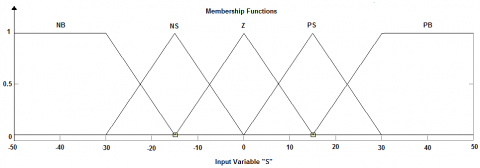Figure 2. S first input membership functions

## 3.png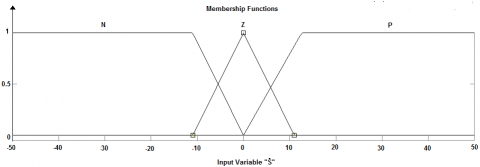Figure 3. $\dot{S}$  second input membership functions

The membership functions of the output K which must be necessary positive are given by the Figure 4.

## 4.png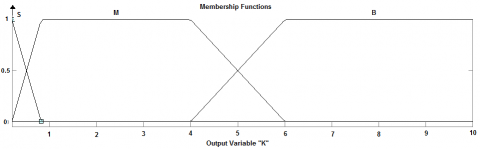Figure 4. K output membership functions

For inference rules, the Mamdani reasoning method is used which is shown in Table 1.

Table 1. Inference rules

 S $\dot{S}$ NB NS Z PS PB N B B S M M Z B M S M B P M M S B B
5. PMSM DTC Fuzzy Sliding System

For PMSM with non-salient pole, the direct and the indirect axis synchronous inductance is equal, namely Ld=Lq=Ls,

The electromagnetic torque can be described as :

$T_{e m}=\frac{3}{2 L_{s}} p\left|\Phi_{r} \| \Phi_{s}\right| \sin \delta$     (17)

where, $\delta$  represents the angle between the stator and rotor flux vectors.

In PMSM, $\left|\Phi_{\mathrm{r}}\right|$  is an invariant . According to the Eq. (16) we notice that it’s possible to control the electromagnetic torque $\mathrm{T}_{\mathrm{em}}$  via adjusting the torque angle $\delta$  with maintaining the of the stator flux linkage amplitude $\left|\Phi_{s}\right|$  without variation.

Using an inverter fed with eight switching modes and six voltage vector plane regions (Figure 5), the stator flux linkage in the stator two-phase reference frame can be given by:

$\Phi_{s}=\int\left(V_{s}-R_{s} i_{s}\right) d t$        (18)

where, $V_{s}$ represents the stator voltage, $I_{s}$ the stator current, and $\mathrm{R}_{s}$ the stator resistance.

The stator flux trajectory is divided into six symmetrical sections (S1 to S6) referring to the inverter voltage vectors, as shown in Figure 5. Stator flux components are used to determine the flux vector position.

## 5.png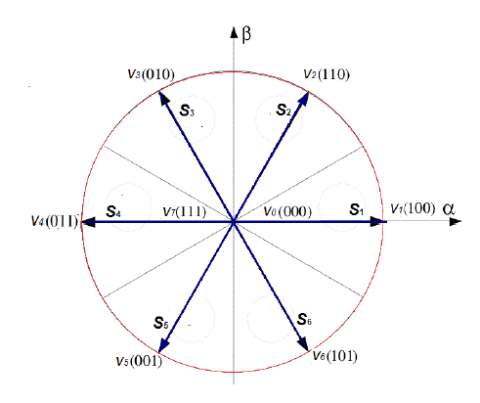Figure 5. Voltage vectors and stator flux sectors

In $(\alpha, \beta)$  frame the estimated flux magnitude and its angular position can be given by:

$\left\|\hat{\phi}_{s}\right\|=\sqrt{\hat{\phi}_{s \alpha}^{2}+\hat{\phi}_{s \beta}^{2}}$       (19)

$\angle \hat{\phi}_{s}=\tan ^{-1}\left(\frac{\widehat{\phi}_{s \beta}}{\widehat{\phi}_{s \alpha}}\right)$         (20)

We can estimate the electromagnetic torque $\mathrm{T}_{\mathrm{em}}$  from the estimated fluxes, and the measured statorique currents, and it can be described as follow:

$\widehat{T}_{e m}=\frac{3}{2} p\left[\widehat{\phi}_{s \alpha} i_{s \beta}-\widehat{\phi}_{s \beta} i_{s \alpha}\right]$         (21)

The flux and torque hysteresis comparators outputs with the flux angular position are used as inputs of a switching table in order to generate the inverter switching sequence .

## 6.png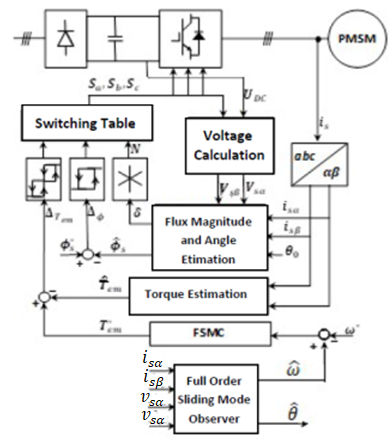Figure 6. DTC- fuzzy sliding mode controller of PMSM with Full order sliding mode observer

The control scheme is presented in Figure 6 where:

$\Delta_{T_{e m}}=\left|T_{e m}^{*}-\widehat{T}_{e m}\right|$          (22)

And

$\Delta_{\phi_{s}}=\left|\phi_{s}^{*}-\hat{\phi}_{s}\right|$             (23)

6. Full Order Sliding Mode Observer of PMSM

If we consider the PMSM model in $(\alpha, \beta)$ frame is given by:

$\left\{\begin{array}{c}\frac{d i_{\alpha}}{d t}=-\frac{R_{s}}{L_{s}} i_{\alpha}+\frac{p K_{e}}{L_{s}} \omega \sin \theta+\frac{1}{L_{s}} u_{\alpha} \\ \frac{d i_{\beta}}{d t}=-\frac{R_{s}}{L_{s}} i_{\beta}+\frac{p K_{e}}{L_{s}} \omega \cos \theta+\frac{1}{L_{s}} u_{\beta} \\ \frac{d \omega}{d t}=\frac{p K_{e}}{J}\left(i_{\beta} \cos \theta-i_{\alpha} \sin \theta\right)-\frac{f}{J} \omega-\frac{1}{J} T_{L} \\ \frac{d \theta}{d t}=\omega\end{array}\right.$              (24)

With:

$\mathrm{u}_{\alpha}, \mathrm{u}_{\beta}:$ Stator voltage in $\alpha-\beta$ axis;

$\mathrm{i}_{\alpha}, \mathrm{i}_{\beta}:$ Stator current in $\alpha-\beta$ axis;

$\mathrm{K}_{\mathrm{e}}$ : Electromotive force;

$\theta$ : Electrical rotor position.

From the machine model given by (23) we can express the Full Order Sliding Mode Observer for PMSM as follow :

$\left\{\begin{array}{c}\frac{d \hat{i}_{\alpha}}{d t}=-\frac{R_{s}}{L_{s}} \hat{i}_{\alpha}+\frac{p K_{e}}{L_{s}} \widehat{\omega} \sin \hat{\theta}+\frac{1}{L_{s}} u_{\alpha} \\ \quad+K_{1} \operatorname{sgn}\left(i_{\alpha}-\hat{i}_{\alpha}\right) \\ \frac{d \hat{i}_{\beta}}{d t}=-\frac{R_{s}}{L_{s}} \hat{i}_{\beta}+\frac{p K_{e}}{L_{s}} \widehat{\omega} \cos \hat{\theta}+\frac{1}{L_{s}} u_{\beta} \\ \quad+K_{1} \operatorname{sgn}\left(i_{\beta}-\hat{i}_{\beta}\right) \\ \frac{d \widehat{\omega}}{d t}=\frac{p K_{e}}{J}\left(\hat{i}_{\beta} \cos \hat{\theta}-\hat{i}_{\alpha} \sin \hat{\theta}\right)-\frac{f}{J} \widehat{\omega}-\frac{1}{J} T_{L} \\ \quad+K_{2} \operatorname{sgn}\left(i_{\alpha}-\hat{i}_{\alpha}\right)+K_{2} \operatorname{sgn}\left(i_{\beta}-\hat{i}_{\beta}\right) \\ \frac{d \hat{\theta}}{d t}=\widehat{\omega}+K_{3} \operatorname{sgn}\left(i_{\alpha}-\hat{i}_{\beta}\right)+K_{3} \operatorname{sgn}\left(i_{\beta}-\hat{i}_{\beta}\right)\end{array}\right.$               (25)

where,

$\hat{\imath}_{\alpha}, \hat{1}_{\beta}$: Estimated stator current value in $\alpha-\beta$ axis;

$\widehat{\omega}$: Estimated speed;

$\hat{\theta}$: Estimated rotor position;

$\mathrm{K}_{1}, \mathrm{~K}_{2}$ and $\mathrm{K}_{3}$ are constant positive gains.

The FO-SMO surfaces are chosen as follow:

$S=\left[\begin{array}{l}S_{\alpha} \\ S_{\beta}\end{array}\right]=\left[\begin{array}{l}i_{\alpha}-\hat{l}_{\alpha} \\ i_{\beta}-\hat{l}_{\beta}\end{array}\right]$                 (26)

Resolving the differential equations system (25) allows obtaining the estimated values of speed and rotor position.

7. Simulation Results

Two different schemes of DTC, the first is DTC with using conventional PI controller and the second is the proposed DTC combined with the fuzzy sliding mode controller.

The simulation is performed in MATLAB/SIMULINK where the PMSM parameters used are presented in Table 2.

Table 2. Parameters of PMSM

 N° Parameter Value 1 Flux linkage 0.175 Wb 2 Number of pole pairs 4 3 Stator resistance 1.4 Ω 4 q-axis inductance 6.62 mH 5 d-axis inductance 6.62 mH 6 Inertia moment 8.10-4kg.m2 7 Viscous friction coefficient 0.0035 N.m.s/rad

Full order sliding mode observer constants used are:

$K_{1}=10^{6}, K_{2}=250$ and $K_{3}=80$.

In order to examine the performance of the proposed control technique, it will be tested first with variations in the reference speed $\omega^{*}$ and then with variations in the load torque $\mathrm{T}_{\mathrm{L}}$ during the simulation period, all that with two different percentages $75 \%$ of $\mathrm{R}_{\mathrm{s}}$ then $125 \%$ of $\mathrm{R}_{\mathrm{s}}$.

7.1 Results with reference speed variations

The speed reference is changed from $\omega^{*}=130 \mathrm{rad} / \mathrm{s}$ to $150 \mathrm{rad} / \mathrm{s}$ at $\mathrm{t}=0.3 \mathrm{~s}$ then to $100 \mathrm{rad} / \mathrm{s}$ at $\mathrm{t}=0.6 \mathrm{~s}$ and finally to $\omega^{*}=70 \mathrm{rad} / \mathrm{s}$ at $t=0.8 \mathrm{~s}$ with a load torque $T_{L}=$ $5 \mathrm{~N} . \mathrm{m}$ applied starting from $t=0.5 \mathrm{~s}$.

## 7.png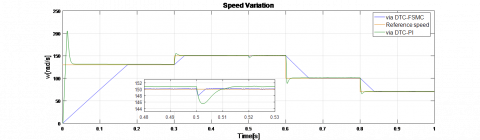Figure 7. Rotation speed with speed reference changes

## 8.png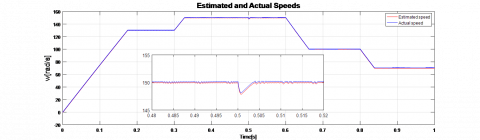Figure 8. Estimated and actual speeds

## 9.png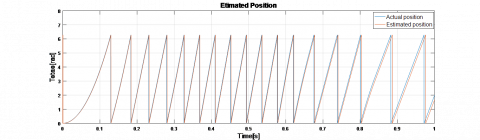Figure 9. Estimated and actual positions

## 10.png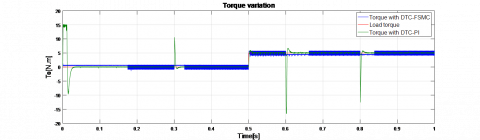Figure 10. Electromagnetic torque via DTC-PI and DTC-FSMC

## 11.png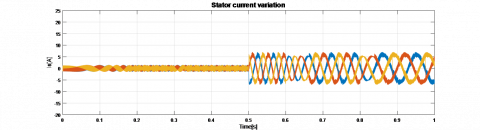Figure 11. Stator current with DTC-FSMC

## 12.png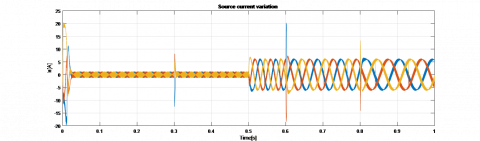Figure 12. Stator current with DTC-PI

With the same simulation parameters except using $75 \%$ of $\mathrm{R}_{\mathrm{s}}$ instead of $\mathrm{R}_{\mathrm{s}}$, the following results are obtained.

## 13.png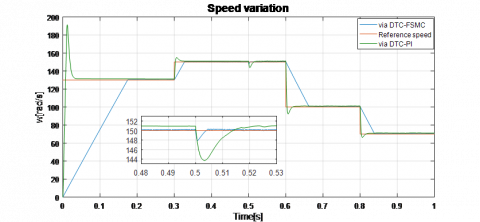Figure 13. Rotation speed with speed reference changes and 75% of Rs

## 14.png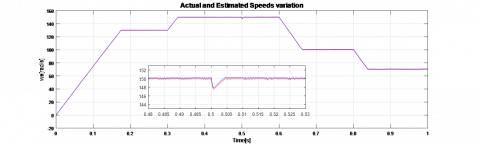Figure 14. Estimated and actual speeds (75% of Rs)

## 15.png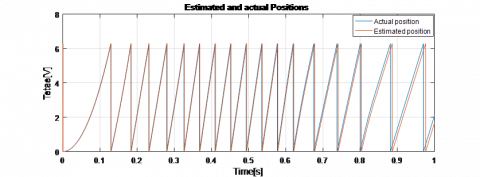Figure 15. Estimated and actual position (75% of Rs)

## 16.png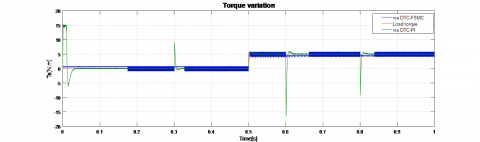Figure 16. Electromagnetic torque via DTC-PI and DTC-FSMC (75% of Rs)

## 17.png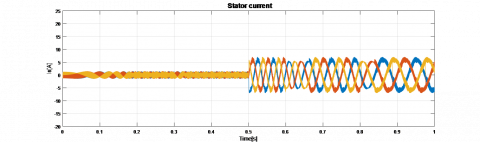Figure 17. Stator current with DTC-FSMC (75% of Rs)

## 18.png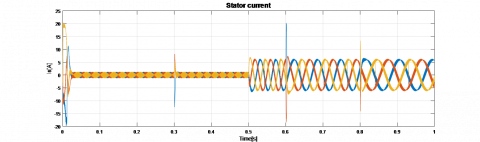Figure 18. Stator current with DTC-PI (75% of Rs)

When the stator armature resistance is increased by 25%, more than its nominal value, the following results are obtained:

## 19.png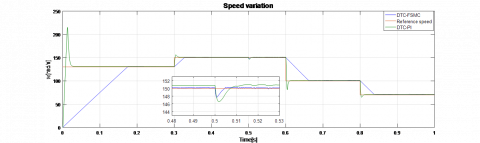Figure 19. Rotation speed with speed reference changes (125% of Rs)

## 20.pngFigure 20. Estimated and actual speeds (125% of Rs)

## 21.pngFigure 21. Estimated and actual positions (125% of Rs)

## 22.pngFigure 22. Electromagnetic torque via DTC-PI and DTC-FSMC (125% of Rs)

## 23.png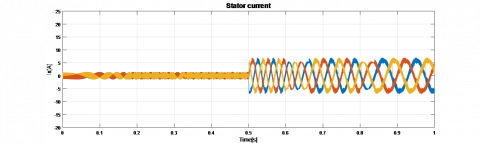Figure 23. Stator current via DTC-FSMC (125% of Rs)

## 24.png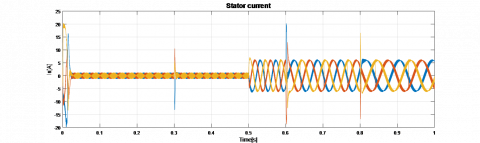Figure 24. Stator current via DTC-PI (125% of Rs)

Figures 7, Figures 13 and Figures 19 show clearly that the behavior of the rotation speed using DTC-FSMC technique in dynamic regime is better compared to the conventional DTC-PI control as well as the electromagnetic torque in Figure 10, Figures 16 and Figures 22, at reference speed change moments.

DTC-FSMC advantage also appears also in stator current variation at reference speed changes moments changes shown by Figures 11, 12, 17, 18, 23 and 24.

The speed error in permanent regime is $\mathrm{e}_{\omega} \simeq 0.05 \mathrm{rad} / \mathrm{s}$ (Figure 7) via DTC-FSMC method, while it reaches $0.8 \mathrm{rad} / \mathrm{s}$ with DTC-PI.

Rotation speed and rotor position are estimated with good accuracy which is shown by Figures 8, 9, 14, 15, 20 and 21.

7.2 Results with load torque variations

Now the load torque is changed from $\mathrm{T}_{\mathrm{L}}=0 \mathrm{~N}$. m. to 5N. $\mathrm{m}$. at $\mathrm{t}=0.3 \mathrm{~s}$ then to $10 \mathrm{~N} . \mathrm{m}$ at $\mathrm{t}=0.6 \mathrm{~s}$ and finally to $2.5 \mathrm{~N} . \mathrm{m}$ at $\mathrm{t}=0.8 \mathrm{~s}$ with setting up the speed reference at $\omega^{*}=$ $130 \mathrm{rad} / \mathrm{s}$.

Figure 25 and Figure 26 show the good reactions of rotation speed and electromagnetic torque using DTC-FSMC compared to DTC with PI controller at load value change moments.

The difference and noticed also in the dynamic regime of the variation of the statoric current in Figures 27 and 28.

## 25.png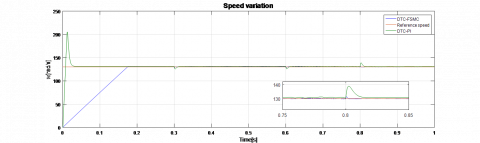Figure 25. Rotation speed with load torque changes

## 26.png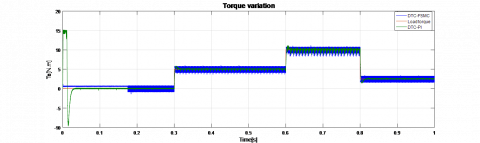Figure 26. Electromagnetic torque with load torque

## 27.png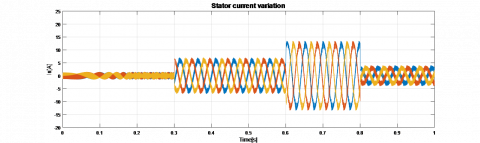Figure 27. Stator current via DTC-FSMC

## 28.png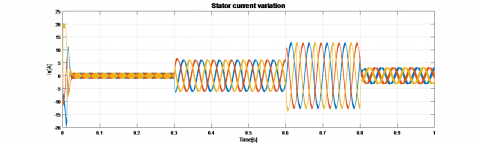Figure 28. Stator current via DTC-PI

All Figures that represent speed and torque gaits show that the dynamic regime using the DT-FSMC control technique is better compared to the conventional DTC-PI, either for speed or electromagnetic torque where overshoots via conventional DTC-PI technique are remarkable.

8. Conclusion

Based on the mathematical model of the permanent magnet synchronous motor and the DTC principle, a rotation speed controller based on a fuzzy sliding mode control technique (FSMC) was designed to replace the PI controller in the conventional DTC. The controller has the advantages of a good robustness against sudden changes in the reference speed values and also the load changes during simulation period.

The robustness of the DTC-FSMC is examined by the stator armature resistance $R_{s}$ changes, where the control technique has demonstrated a good stability in speed and torque responses especially in the dynamic regime with the remarkable absence of overshoots in the speed and electromagnetic torque variations during sudden change of reference rotation speed.

The DTC-FSMC scheme also has given an improved precision in permanent regime in comparison with the conventional DTC-PI technique.

Full Order Sliding Mode Observer used with the proposed DTC-FSMC has proven a good accuracy in speed and rotor position estimation.

Nomenclature
 $\mathrm{R}_{\mathrm{s}}$ Stator armature resistance, Ω $\mathrm{L}_{\mathrm{d}}, \mathrm{L}_{\mathrm{q}}$ Direct and quadrature inductance, H $\omega$ Rotor angular speed thermal, rad.s-1 p Pole pairs number $\mathrm{u}_{\mathrm{d}}, \mathrm{u}_{\mathrm{q}}$ Stator voltage in d-q-axis, volts $\mathrm{i}_{\mathrm{d}}, \mathrm{i}_{\mathrm{q}}$ Stator current in d-q-axis, A $\Phi_{\mathrm{f}}$ Flux created by the rotor magnets, Wb $\mathrm{T}_{\mathrm{em}}$, $\mathrm{T}_{\mathrm{L}}$ Electromagnetic and load torques, N.m $\mathrm{F}_{\mathrm{c}}$ Viscous friction coefficient, N.m.s.rad-1 J Total moment of inertia of the motor and load, Kg.m2
References

 Hooshyar, H., Savaghebi, M., Vahedi, A. (2007). Synchronous generator: Past, present and future. IEEE-AFRICON Conference, Windhoek, South Africa, pp. 1-7. https://doi.org/10.1109/AFRCON.2007.4401482

 Sun, D., Fang, W.Z., He, Y.K. (2001). Study on the direct torque control of permanent magnet synchronous motor drives. IEEE-ICEMS Conference, Shenyang, China, 1: 571-574. https://doi.org/10.1109/ICEMS.2001.970740

 Guo, R., Wang, X., Zhao, J., Yu, W. (2011). Fuzzy sliding mode direct torque control for PMSM. Eighth International Conference on Fuzzy Systems and Knowledge Discovery, Shanghai, China, pp. 511-514. https://doi.org/10.1109/FSKD.2011.6019599

 Wang, H., Li, S., Yang, J., Zhou, X. (2016). Continuous sliding mode control for permanent magnet synchronous motor speed regulation systems under time-varying disturbances. Journal of Power Electronics, 16(4): 1324-1335. https://doi.org/10.6113/JPE.2016.16.4.1324

 Walambe, R.A., Joshi, V.A. (2018). Closed loop stability of a PMSM-EKF controller-observer structure. IFAC-PapersOnLine, 51(1): 249-254. https://doi.org/10.1016/j.ifacol.2018.05.062

 Bakhti, I., Chaouch, S., Makouf, A., Douadi,T. (2016). Robust sensorless sliding mode control with Luenberger observer design applied to permanent magnet synchronous motor. 5th International Conference on Systems and Control (ICSC), pp. 204-210. https://doi.org/10.1109/ICoSC.2016.7507051

 Attou, A., Massoum, A., Chiali, E. (2013). Sliding mode control of a permanent magnets synchronous machine. IEEE-INSPEC Conference, Istanbul, Turkey, pp. 115-119. https://doi.org/10.1109/PowerEng.2013.6635591

 Zhao, Y., Huang, Z. (2015). Fuzzy direct torque control of permanent magnet synchronous motors. 2015 12th International Conference on Fuzzy Systems and Knowledge Discovery (FSKD), pp. 330-334. https://doi.org/10.1109/FSKD.2015.7381963

 Sun, D., He, Y.K., Zhu, J.G. (2004). Sensorless direct torque control for permanent magnet synchronous motor based on fuzzy logic. The 4th International Power Electronics and Motion Control Conference, 3: 1286-1291.

 Lu, Z., Sheng, H., Hess, H.L., Buck, K.M. (2005). The modeling and simulation of a permanent magnet synchronous motor with direct torque control based on Matlab/Simulink. IEEE International Conference on Electric Machines and Drives, San Antonio, TX, USA. https://doi.org/10.1109/IEMDC.2005.195866

 Saadaoui, O., Khlaief, A., Abassi, M., Chaari, A., Boussak, M. (2016). Sensorless FOC of PMSM drives based on full order SMO. International Conference on Sciences and Techniques of Automatic Control and Computer Engineering (STA), pp. 663-668. https://doi.org/10.1109/STA.2016.7952106